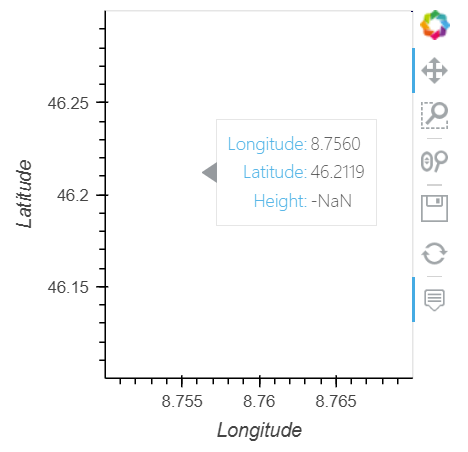# One dimension missing on hover after rasterize point data

Hi, I am trying to make scatter plot for large data with `holoviews.operation.datashader.rasterize` and show the hover. But I find one dimension of the data is missed on the hover after I use the `rasterize`. Here is the minimal example code:

``````import numpy as np
import geoviews as gv
gv.extension('bokeh')

lon = np.array([8.75,8.76,8.77])
lat = np.array([46.1,46.2,46.3])
height = np.array([0.0,0.1,0.2])
index = np.array([0,1,2])

points = gv.Points(np.stack((lon,lat,height,index),axis=-1),
kdims=['Longitude','Latitude'],vdims=['Height','Index'])
points.opts(tools=['hover'])
``````

The result looks perfect, `Longitude`, `Latitude`, `Height` and `Index` are all showed. (sorry I can only upload one image as a new user on this website. So, I upload the most important one.)
The I rasterize it:

``````points = rasterize(points,aggregator=datashader.mean('Height'),vdim_prefix='',precompute=True)
points.opts(tools=['hover'])
``````The `Index` dimension is missing on the hover.

Then I try to print the rasterized `points`:

``````print(points)
``````

and get:

``````:DynamicMap   []
:Image   [Longitude,Latitude]   (Height)
``````

It looks like the fourth dimension is missed. I have searched the overall manual but didn’t find anything related to it. Please let me know if anybody knows how to preserve this `Index` dimension and show it on the hover. Thanks a lot!

Hi @kanglcn and thanks for taking the time to write down a small code to illustrate what you are after.
The following snippet uses `inspect` from `holoviews.operations.datashader`. It is designed to give back the hover functionnality that is otherwise lost with the aggregation that datashader does (it is not much documented for now so you can have a look at Jean-Luc Stevens: Seeing the needle AND the haystack: single-datapoint selection for billion-poin... - YouTube, starting at 13m for some details on `inspect`, but the whole video is really worth watching).

• I put you data in a dataframe to make things cleaner and also because I tried to achieve the hover through hvplot.pandas (without success).
• The dynspread is here to make datashader points more visible, so things are easier for the hover inspection.
• The code does not work with `gvpoints`. Indeed, the points disappear as soon as the mouse enters the frame. This looks like a bug.
• For more inspiration on `inspect`, have a look at the AIS demo which is extremely good.
``````import numpy as np
import pandas as pd
import holoviews as hv
#import geoviews as gv
hv.extension('bokeh')

lon = np.array([8.75,8.76,8.77])
lat = np.array([46.1,46.2,46.3])
height = np.array([0.0,0.1,0.2])
index = np.array([0,1,2])

df = pd.DataFrame(dict(Longitude=lon,Latitude=lat,Height=height,Index=index))

#gvpoints = gv.Points(df, ['Longitude','Latitude'])
hvpoints = hv.Points(df, ['Longitude', 'Latitude'])